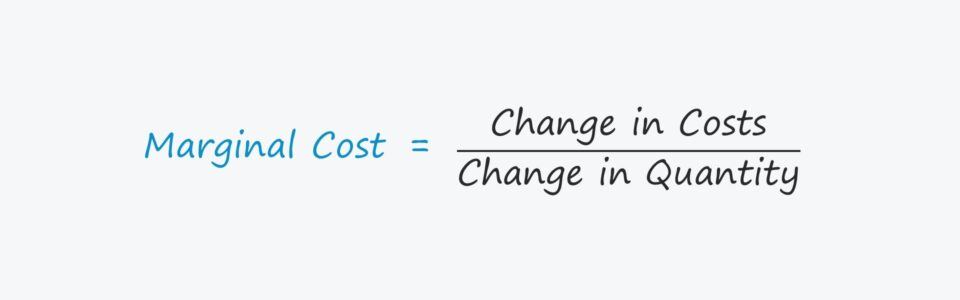# Marginal Cost

Understand the Concept of Marginal Costs• What does the marginal cost measure?
• What is the formula used to calculate the marginal cost?
• If a company’s marginal costs are plotted on a graph, which shape is created?
• What is the purpose of calculating the marginal cost?

## Marginal Cost Analysis

The marginal cost of production captures the additional cost of producing one more unit of a good/service.

The marginal cost is fundamental to companies being able to price goods and services appropriately and turning a profit.

The costs of operating a company can be categorized as either fixed or variable costs.

• Fixed Costs: Costs that remain constant regardless of production volume and sales performance (e.g. rent, utilities)
• Variable Costs: Costs that fluctuate based on the company’s production output and sales figures (e.g. raw materials, direct labor)

If changes in the production volume result in total costs changing, the difference is mostly attributable to variable costs.

Analysis of the marginal cost helps determine the “optimal” production quantity, where the cost of producing an additional unit is at its lowest point.

The marginal cost of production must be lower than the price per unit for a company to be profitable – thus, the marginal cost pinpoints the output volume and pricing where incremental costs are reduced.

## Marginal Cost Graph & Economies of Scale

Marginal costs provide insights into the optimal production output and pricing, i.e. the point where economies of scale are achieved.

Beyond the optimal production level, companies run the risk of diseconomies of scale, which is where the cost efficiencies from increased volume fade (and become negative).

If marginal costs are plotted on a graph, the curve would be “U-shaped,” as costs gradually shift downward once production volume increases.

But eventually, the curve reverses trajectory and climbs upwards due to the law of diminishing marginal returns.

Understanding the relationship between changes in quantity and changes in costs results in informed decisions when setting production targets.

Companies operating near their optimal production level improve the odds of a net positive impact on cash flows and profit margins.

## Marginal Cost Formula

The marginal cost formula requires three inputs:

1. Total Costs of Production
2. Change in Costs
3. Change in Quantity

The first step is to calculate the total cost of production by adding the total fixed costs to the total variable costs.

###### Total Costs Formula
• Total Costs = Total Fixed Costs + Total Variable Costs

Next, the change in total costs and change in quantity (i.e. production volume) must be tracked across a specified period.

The final step is to calculate the marginal cost by dividing the change in total costs by the change in quantity.

###### Marginal Cost Formula
• Marginal Cost = (Change in Costs) / (Change in Quantity)

## Marginal Cost Example Calculation

Suppose a company produced 100 units and incurred total costs of \$20k.

• Year 1 Costs = \$20k
• Year 1 Quantity = 100 Units

In the following year, the company produces 200 units at a total cost of \$25k.

• Year 2 Costs = \$25k
• Year 2 Quantity = 200 Units

The total change in cost is \$5k, while the total change in production is 100 units.

• Marginal Cost = (\$30k – \$25k) / (200 – 100)
• Marginal Cost = \$50

In our illustrative example, the marginal cost of production comes out to \$50 per unit.Inline FeedbacksLearn Financial Modeling Online

Everything you need to master financial and valuation modeling: 3-Statement Modeling, DCF, Comps, M&A and LBO.

X

The Wall Street Prep Quicklesson Series

7 Free Financial Modeling Lessons

Get instant access to video lessons taught by experienced investment bankers. Learn financial statement modeling, DCF, M&A, LBO, Comps and Excel shortcuts.